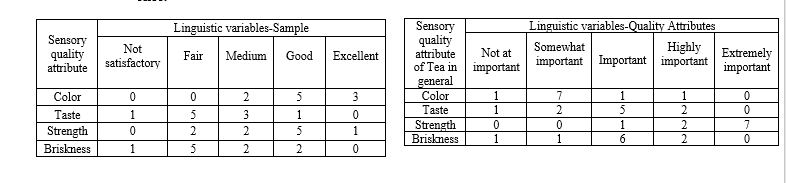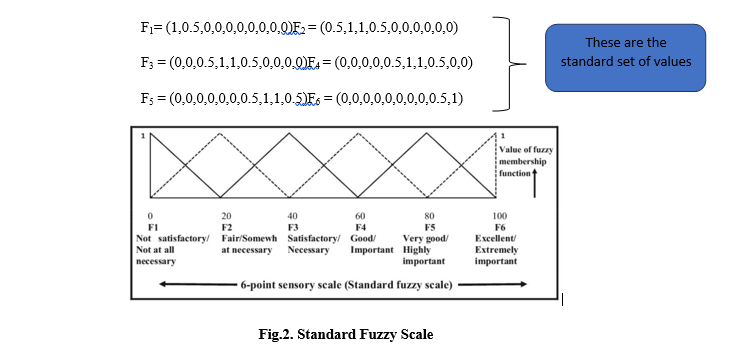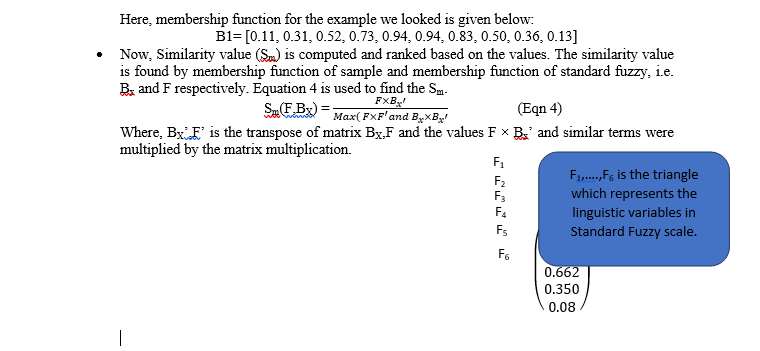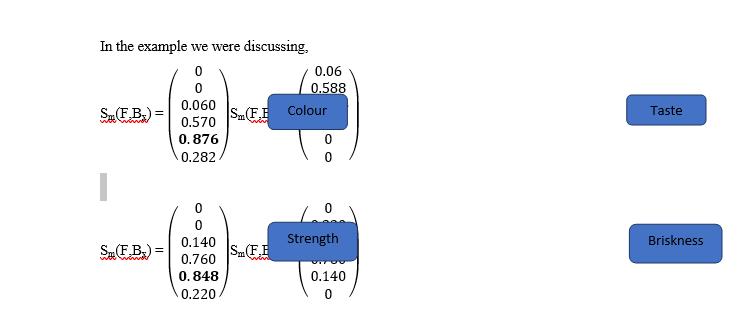+918130603114 info@fmtmagazine.in
Select Page

## Beyond Pizza at Brik Oven

By G.Vigneshwaran, Muhammad Salman Chukkan, Nachal N

Introduction

Sensory evaluation in simple terms can be defined as a ‘Scientific discipline dealing with the sensorial perception of a food’. Sensory evaluation gives major insights in NPD and reformulation to get the best recipe or product. The most common practice of sensory evaluation is based on 9-point Hedonic Scale. The major disadvantage is that in this method the importance of quality attributes is not taken into consideration and it is also found that a decision making is not essentially easy. That’s why Fuzzy logic approach comes to limelight. Because, through Fuzzy logic approach we can interpret the linguistic inputs from the evaluators and also it is a great tool for making vice decision.

Fuzzy logic is an algorithm that converts subjective data into objective data and then quantified. In this method, the evaluator is asked to record their observation in the table of the linguistic variables as shown below see table 1, which demonstrates their perception about different quality attributes of the given sample and as well as evaluator’s perception on general attributes of the food product see table 2. Later the count is taken for each attribute with the linguistic variable. A linguistic variable means any word or sentence describing the sense or quality attributes by the evaluator.

Table 1: Sensory evaluation of Food sample Table 2: Preference on the importance of quality attributesSensory scale is divided to represent the linguistic variable on linear scale. The linguistic variable is plotted on the scale in an interval of [0,100]. Membership function can be simply defined as the degree to which the inputs belong to a set. The linguistic variable in the triangular membership function is represented as a triplet (a,b,c). Here the term “a” represents the coordinate of the abscissa and the number “b” “c” of the triplet represents the distance to the left and right, respectively, of the first number “a” (Fig.2). It is to be noted that, the value of the term in the triplets has to lie in the interval [0, 100] if not they are ignored, as they violate the triangular membership function. For example, take fair, then the triplet would be (25,25,25) and excellent would be (100,25,0) from Fig.1

General guidelines to perform Sensory evaluation through Fuzzy Logic with example.

• Brief the team about the product. Selected the best five attributes related the product, which are true representative of the product. Let’s assume we have 10 judges and the test is done for Tea.
• Now get the sensory attributes recorded in a 9-Point hedonic scale.
• Now, convert the values from 9-point hedonic scale into 5 point linguistic variable. For example, A rating of 1 and 2 in hedonic scale can be converted as Not satisfactory in 5 point linguistic scale. The rational to do so is entirely depended on the leader who leads the sensory evaluation. And add in the Table 1.
• Now get the relative importance for the selected quality attributes from the judges. And in the table 2. A number in a junction of column and row is number of judges with same judgment. For example, there are 2 judges saying that color of the sample is medium. Table 1 is just for a sample likewise multiple tables shall be formed as required. Whereas Table 2 is just made once.• Now convert the linguistic variables of into triplets. As given here, not satisfactory would be (0,0,25), Fair (25,25,25) etc. This can be derived by looking into Fig.1.
• Now, Obtain the triplet of each and every individual sample. The calculation is as follows. The same shall be done for all the attributes related to sample. The triplet related to Taste of sample would be addressed as ST. (Table 3). Same way, ST=(35.00 22.50 25.00), SS=(62.50 25.00 22.50), SB=(37.50, 22.50 25.00).(SC, ST, SB, SS are termed as Sample attribute)

SC=  = (77.50 25.00 17.50)

• Now, obtain the triplet of each and every Quality attributes. The calculation is as follows. The same shall be obtained for other attributes too. The triplet of Taste of quality attribute would be addressed as QT. Same way QT=(45.00 22.50 25.00), QS=(90.00 25.00 7.50), QB=(47.50,22.50, 25.00). (QC, QT, QS, QB are termed as Quality attribute)

QC= =(30.00 22.50 25.00)

• Now the Relative Quality Attribute is found from Quality attribute. For this add all the first number of the triplets of various quality attribute. Same way QTRelative=(0.21 0.11 0.12), QSRelative=(0.42 0.12 0.04), QBRelative = (0.22 0.11 0.12)

Qsum= Sum of the first number of triplet (QC, QT, QS, QB)

Qsum=(30+45+90+47.5) = 212.5

QCRelative =  = (0.14 0.11 0.12)

• Now find the overall sensory score (SO) is found by the following equation. The triplet addition (Eqn 2) and multiplication (Eqn 1) rules as given below. By using this rule the Overall sensory score is found.

(a b c) .(d e f) = (a × d a × e + d × b   a × f + d × c)  (Eqn 1)

(a b c) + (d e f) = (a+d b+e c+f)                        (Eqn 2)

SO = SC*QCrel + ST*QTrel + SS*QSrel + SB*QBrel

SO  = (52.70 47.98 43.15)

• Now estimation of membership function (F) of Standard Fuzzy. To obtain the sensory score with more impression, we convert the 5-point sensory scale to 6-point sensory scale. In Fig.2. F1, F2, F3, F4, F5 and F6represents the sensory scales which follows the triangular distribution pattern and membership function is applied. In fact, the maximum value of membership function is 1. The value of F1 through F6 is represented by standard set of 10 values.• Now, Overall membership function of sample on standard scale is computed. The membership function of sample (Bx) on standard fuzzy scale (6-Point scale). The membership function of sample is required to calculate similarity value (Sm). The estimation is done by the conditions given below:

Triplets associated with SO of the sample and any value ‘x’ in abscissa of standard fuzzy scale, valueof membership function Bcan be expressed as:

Bx =  for (a-b) < x < a

Bx =  for (a)< x < (a+c)                              (Eqn 3)

Bx = 0 for x< (a-b) or x > (a+c)

For each sample and its triplets, the value of membership function Bx at x = 0, 10, 20, 30, 40, 50, 60, 70, 80, 90, and 100 can be found out from Eq. 3. This membership function value of sample (Bx) on standard fuzzy scale will be given a set of ten numbers which are “(maximum value of Bx at 0<x<10), (maximum value of Bx at 10<x<20), (maximum value of Bx at 20<x<30), (maximum value of Bx at 30<x< 40), (maximum value of Bx at 40 < x < 50), (maximum value of Bx at 50<x<60), (maximum value of Bat 60<x<70), (maximum value of Bx at 70<x<80), (maximum value of Bx at 80<x<90),(maximum value of Bx at 90<x<100).”Here, in the similarity value of a sample we identify the how the sample is rated by looking which is the largest value in the matrix. In the example, 0.662 which means it is F4. I.e. the sample is good.

• Now, Quality is to be ranked. This is also done by the similarity values. In the same manner we done the above. i) That is the triplet of the sample attributes are taken (i.e. SC, ST, SS, SB). ii)Then the Membership function of fuzzy scale is found (F). iii) Then the membership function of sample is found as explained above (Bx­). iv) Then the similarity value is found as explained above.

This exercise is basically done to understand which sensory parameter is the most important. And this insight can be then used to make wonderful New Products. Which can be quite successful.Thus, it is found that Colour is the most important quality attribute. Which is followed by the Taste of the Tea and then Strength and Briskness.

(Note: Here, we only took one sample in order to simplify the explanation. Typically, there would be multiple samples. The method seems to difficult but if it is worked out once it would be easy. It is recommended to do all sort of calculation in excel to save time.)

Conclusion:

Fuzzy logic based sensory evaluation can be a great tool for decision making. This method removes the vagueness of the traditional sensory evaluation. Also, by implementing we get a great insight about the important quality attributes which can be very helpful in the NPD process.

Flowchart: A flowchart is given below to summarise the process.